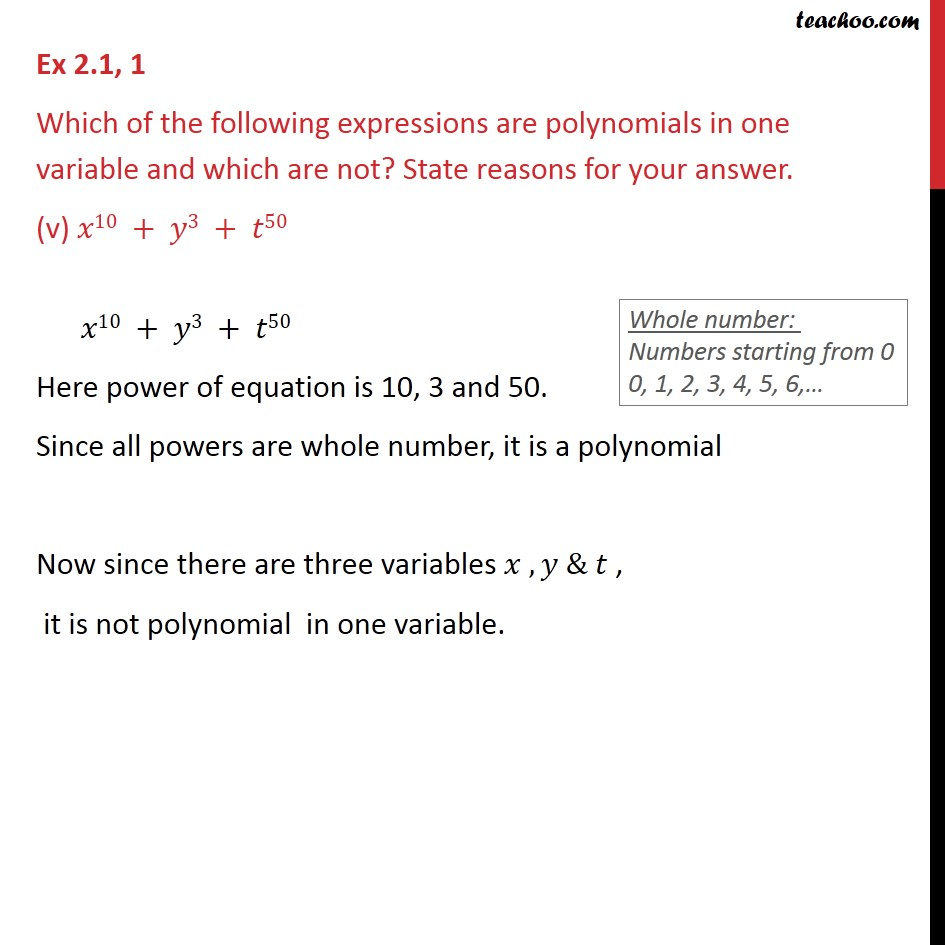Ex 2.1

Chapter 2 Class 9 Polynomials
Serial order wiseGet live Maths 1-on-1 Classs - Class 6 to 12

### Transcript

Ex 2.1, 1 Which of the following expressions are polynomials in one variable and which are not? State reasons for your answer. (v) 𝑥10 + 𝑦3 + 𝑡50 𝑥10 + 𝑦3 + 𝑡50 Here power of equation is 10, 3 and 50. Since all powers are whole number, it is a polynomial Now since there are three variables 𝑥 , 𝑦 & 𝑡 , it is not polynomial in one variable.